﻿ Digital Circuits and Systems - Circuits i Sistemes Digitals (CSD) - EETAC - UPCBachelor's Degree in Telecommunications Systems and in Network Engineering## Comp_4bit plan C2: structural hierarchical multiple-file VHDL

Structural design of a 4-bit expandable comparator

1. Specifications

Design a 4-bit expandable comparator Comb_4bit using plan C2: hierarchical structure based on components of the same kind, for instance Comp_1bit and logic gates if necessary.

Comp_4bit has the symbol and truth table represented in Fig. 1.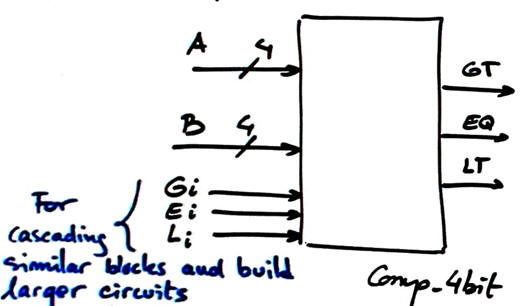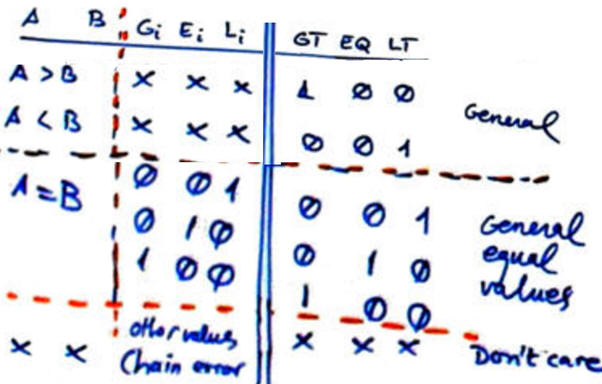Fig. 1. Symbol and truth table of a 4-bit comparator with extra signals for expandability.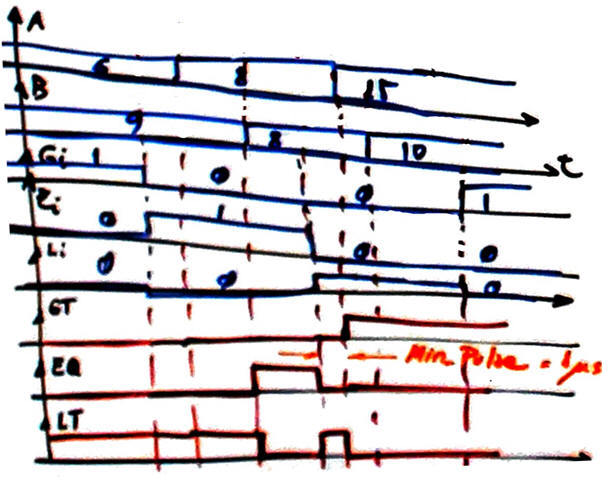Fig. 2. Example of timing diagram. Outputs are deduced from the circuit's truth table.

2. Planning

Plan C2. Structural hierarchical, a plan based on interconnecting or chaining components (circuit expansion) and extra logic when necessary. For instance, Fig. 3 is a plan using Comp_1bit components and signals.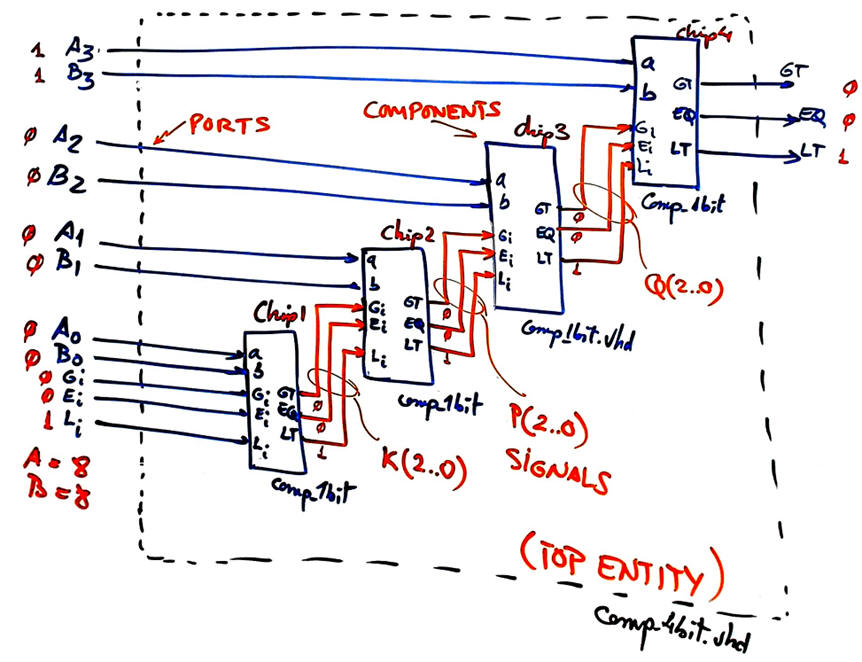Fig. 3. Example plan of Comp_4bit fully annotated, ready for VHDL translation.

Project location:

C:\CSD\P3\Comp_4bitC2\(files...)

Note: The same component Comp_4bit is designed using plan A equations. You can compare solutions. Which is faster? Which is using more PLD resources (logic elements)?

3. Developing the project using EDA tools

This is the 4-bit comparator Comp_4bit.vhd that corresponds exactly to the sketch in Fig. 3.

Get the component  Comp_1bit that is used up to 4 times from tutorials or plan B or . Any of them is perfectly valid. Note: if you use Comp_1bit from plan C2, other components such MUX_2, MUX_4 and MUX_8 will become part of this project too, enlarging the hierarchy with more layers.

Run a EDA project to synthesise the circuit. Select a target chip like Intel Cyclone IV EP4CE115F29C7.

Fig. 4 shows an example of RTL schematic generated after the synthesis process.Fig. 4. RTL view from Intel Quartus Prime.

Fig. 5 shows how the circuit is synthesised using Cyclone IV logic resources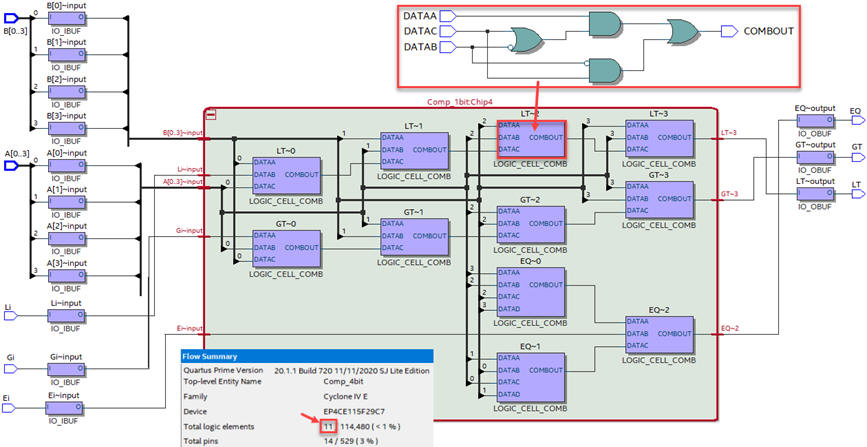Fig. 5. Example technology view for a Cyclone IV FPGA chip.

How many resources (logic elements) are used? As it can be seenfrom the tecnology schematic or fron the project summary, 11 logic cells are used.

4. Testing and validating the design

Test bench schematic for validating our design is always the same: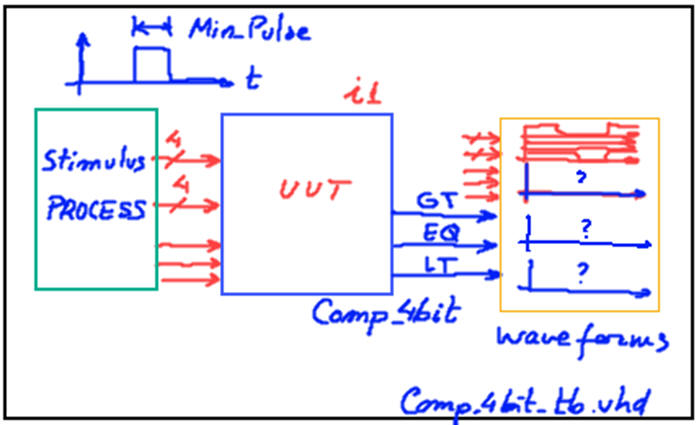Fig. 6. Testbench fixture.

Fig. 2 timing diagram can be translated to a VHDL testbench process. An example test bench Comp_4bit_tb.vhd from where to copy and adapt vector stimulus input signals and constant Min_Pulse.

Run the VHDL simulation EDA tool to obtain and discuss the timing diagram.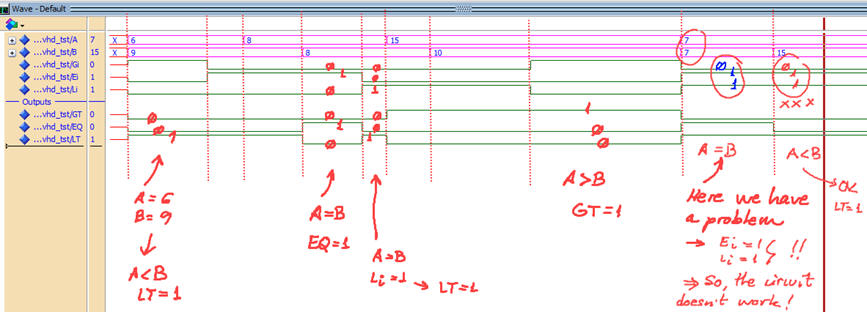Fig. 7. Example testbench showing ideal results. The same results and discussion for the Comp_4bit invented using plan A.

5. Testing at the gate-level the technology schematic to measure circuit's speed

Using a gete-level simulation is possible to measure propagation delays at a given signal transition. How long does it take to propagate signals from a given switching input to an output? For example in Fig. 8 we can observe A = B = "1000", Ei = '1', and at a give time, Li = '1' and Ei = '0'. The new outputs take 8.36 ns to be stable.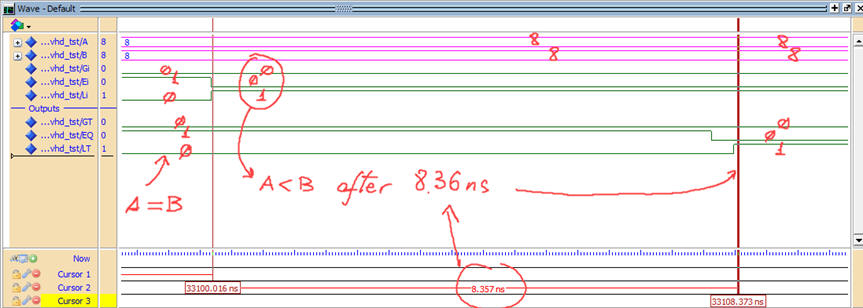Fig. 8. Example testbench showing real results in a specific signal transition.

Using the timer analyser tool is possible to measure the worst-case propagation time scenario, and thus determine  the maximum circuit's speed for processing comparisons.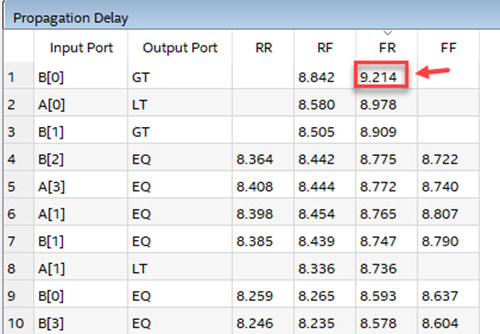Fig. 9. Timing analyser estimating the worst-case scenario on propagation delay.

The longest path is established from input B(0) to output GT. When B(0) is switching from high to low (fall) and GT is switching from low to high (rise), it takes tP =  9.214 ns to generate the correct output. 109 millions of 4-bit comparisons per second.

The final implementation of this 4-bit chained comparator seems to be slightly faster than the same circuit Comp_4bit designed using plan A. Therefore, comparisons on speed and performance must be done studying larger comparators.

6. Report

At least five handwritten sheets of paper as usual in CSD.  Follow this for writing reports.

7. Prototyping

Use training boards and perform laboratory measurements to verify how the circuit works.